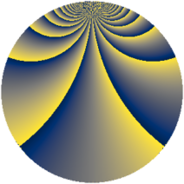# Properties

 Label 2736.3.fqLevel $2736$ Weight $3$ Character orbit 2736.fq Rep. character $\chi_{2736}(367,\cdot)$ Character field $\Q(\zeta_{18})$ Dimension $1440$ Sturm bound $1440$

# Related objects

## Defining parameters

 Level: $$N$$ $$=$$ $$2736 = 2^{4} \cdot 3^{2} \cdot 19$$ Weight: $$k$$ $$=$$ $$3$$ Character orbit: $$[\chi]$$ $$=$$ 2736.fq (of order $$18$$ and degree $$6$$) Character conductor: $$\operatorname{cond}(\chi)$$ $$=$$ $$684$$ Character field: $$\Q(\zeta_{18})$$ Sturm bound: $$1440$$

## Dimensions

The following table gives the dimensions of various subspaces of $$M_{3}(2736, [\chi])$$.

Total New Old
Modular forms 5832 1440 4392
Cusp forms 5688 1440 4248
Eisenstein series 144 0 144

## Trace form

 $$1440q + 72q^{9} + O(q^{10})$$ $$1440q + 72q^{9} + 360q^{33} + 216q^{41} + 5040q^{49} - 216q^{73} - 504q^{81} - 1080q^{97} + O(q^{100})$$

## Decomposition of $$S_{3}^{\mathrm{new}}(2736, [\chi])$$ into newform subspaces

The newforms in this space have not yet been added to the LMFDB.

## Decomposition of $$S_{3}^{\mathrm{old}}(2736, [\chi])$$ into lower level spaces

$$S_{3}^{\mathrm{old}}(2736, [\chi]) \cong$$ $$S_{3}^{\mathrm{new}}(684, [\chi])$$$$^{\oplus 3}$$